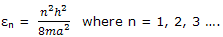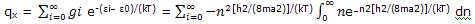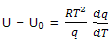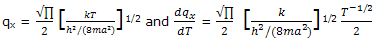Theory of one dimensional motion

For motion in one dimension, the distribution of the molecules over quantum states, speeds, and energies can be deduced.

Here we show that the energy of a macroscopic gas sample can be described on the basis of our knowledge of the quantum states allowed to the molecules of the gas and the distribution expressed by the Boltzmann expression. We begin by studying the translational motion in one dimension of a collection of molecules. You will see how the procedure is fascillated by the partition function.

Partition function: the molecules of a gas that move along one dimension can have, any of the energies given byFor gas samples we can assume a cubic container and express a as V1/3, where V is the volume of the sample.

The partition function for one-dimensional translational motion can be developed by recognizing that

The translational energy of the lowest-energy state is small compared with the energies of most of the populated states and can be set equal to zero.

The translational-energy spacing between successive energy levels is small compared with the range of energies of the populated states.

The degeneracy of each energy level is unity.

On this basis, the partition function summation over the translational energies can be replaced by integration, and the partition function is expressed asThe integral is one of the definite integrals dealt by using the general result shown there, we obtainExample: calculate the partition function for the translational motion of N2 molecules free to move along one dimension of a 1-L cubic container. The temperature is 25°C.

Solution: the translational-energy factor h2/(8ma2) can be calculated conveniently from the expression of this equation. The mass of M of 1 mol of N2 molecules is 0.02801 kg, and V = 1 L = 10-3 m3. Thus= 1.180 × 10-40 J

The value of kT, to which the energy spacing factor is compared, is

kT = (1.3807 × 10-23 J K-1) (298.15 K) = 4.116 × 10-21 J

The partition function is calculated asthis large partition function value indicates that very many states are available to the molecules. This result, in the calculations, from the smallness of h2/(8ma2compared to kT.

Average energy: the one dimensional translational energy of 1 mol of gas molecules can now be deduced. The general thermal-energy expression isThe partition function for one-dimensional translational motion givessubstitution of the equation expressions in the equation for U - U0 gives

U - U0 = ½ RT

We have come by this long route to the result that we obtained from the simple classical kinetic-molecular theory. The translational energy per degree of freedom is ½ RT

#### Related Questions in Chemistry

• ##### Q :Colligative effect Choose the right

Choose the right answer from following. Which one of the statements written below concerning properties of solutions, explain a colligative effect: (a) Boiling point of pure water decreases by the addition of ethano (b) Vapour pressure of pure water d

• ##### Q :Symmetry Elements The symmetry of the

The symmetry of the molecules can be described in terms of electrons of symmetry and the corresponding symmetry operations.

Clearly some molecules, like H2O and CH4, are symmetric. Now w

• ##### Q :Direction of dipole moment expected

Illustrate the direction of the dipole moment expected for hydrogen bromide?

• ##### Q :Product of HCl Zn Illustrate  the

Illustrate  the product of HCl Zn?

• ##### Q :Acid Solutions Choose the right answer

Choose the right answer from following. Volume of water needed to mix with 10 ml 10N NHO3 to get 0.1 N HNO3: (a) 1000 ml (b) 990 ml (c) 1010 ml (d) 10 ml

• ##### Q :Determining highest normality What is

What is the correct answer. Which of the given solutions contains highest normality: (i) 8 gm of KOH/litre (ii) N phosphoric acid (iii) 6 gm of NaOH /100 ml (iv) 0.5M H2SO4

• ##### Q :Concentration of Sodium chloride

Provide solution of this question. If 25 ml of 0.25 M NaCl solution is diluted with water to a volume of 500ml the new concentration of the solution is : (a) 0.167 M (b) 0.0125 M (c) 0.833 M (d) 0.0167 M

• ##### Q :Molarity of Sodium hydroxide Select the

Select the right answer of the question. Molarity of 4% NaOH solution is : (a) 0.1M (b) 0.5M (c) 0.01M (d) 0.05M

• ##### Q :Relative reactivity Which is more

Which is more reactive towards nucleophilic substitution aryl halide or vinyl halides

• ##### Q :Mole fraction and Molality Select the

Select the right answer of the following question.What does not change on changing temperature : (a) Mole fraction (b) Normality (c) Molality (d) None of these